To lowest order in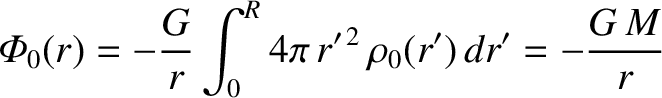, the moment of inertia of our body about its rotation axis can be written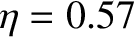(D.43)

It follows from Equation (D.27) that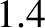(D.44)

where we have integrated by parts. Now, Equation (D.42) implies that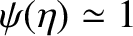(D.45)

where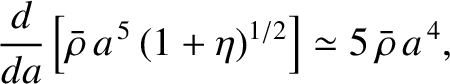. Hence, we obtain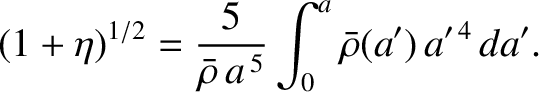(D.46)

It is convenient to define the dimensionless moment of inertia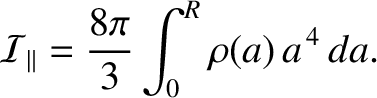(D.47)

Making use of Equations (D.29) and (D.46), we deduce that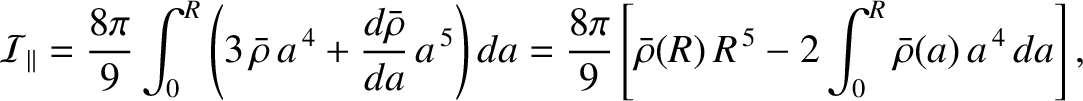(D.48)

However, Equation (D.31) yields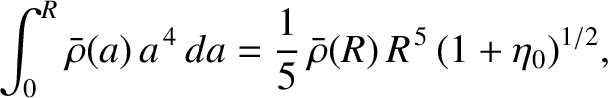(D.49)

where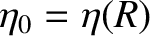. Hence, we obtain the so-called Darwin-Radau equation (Radau 1885; Darwin 1899),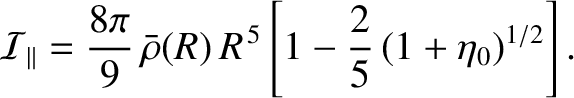(D.50)

This equation relates the degree of flattening of an inhomogeneous rotating body to its rotational angular velocity and its moment of inertia about its rotation axis. The Darwin-Radau equation is only valid if the response of the body to the centrifugal potential is fluid-like. Recall, also, that the equation was derived under the assumption that the rotation is sufficiently slow thatand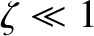can both be treated as small parameters, and, in particular, that any quantities that are second order, or higher, in these parameters can be neglected in the analysis. Note, finally, that the derivation of the Darwin-Radau equation depends crucially on the approximation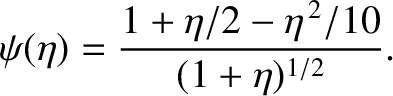, where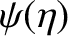is defined in Equation (D.40).

For a rotating body in hydrostatic equilibrium (in the co-rotating frame), Equation (6.43) yields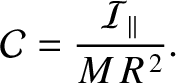(D.51)

where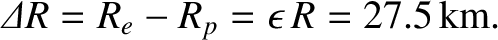is the body's dimensionless gravitational quadrupole moment. [See Equation (8.86).] The previous two equations can be combined to give the following alternative versions of the Darwin-Radau equation: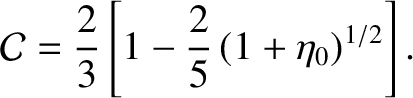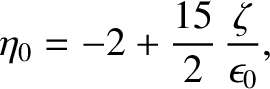(D.52) and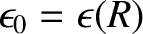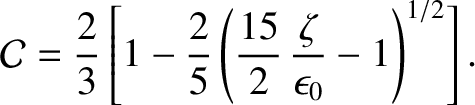(D.53)